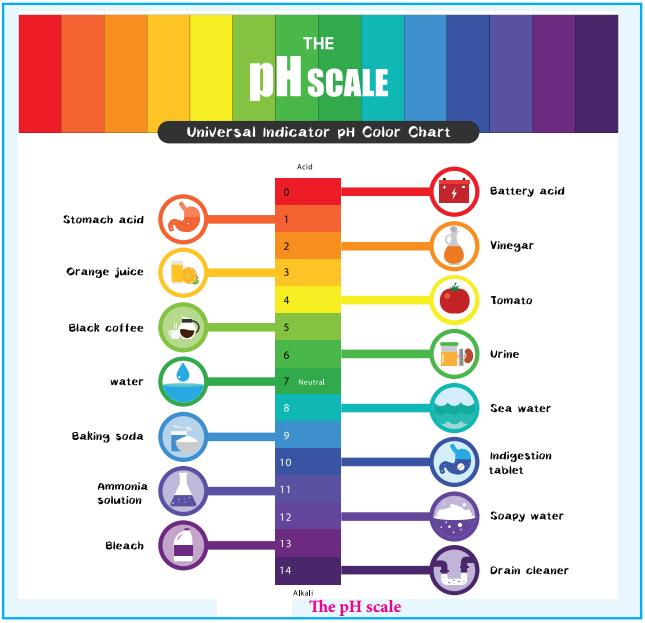Find free online Chemistry Topics covering a broad range of concepts from research institutes around the world.

## The pH Scale

We usually deal with acid / base solution in the concentration range 10-1 to 10-2M. To express the strength of such low concentrations, Sorensen introduced a logarithmic scale known as the pH scale. The term pH is derived from the French word ‘Purissance de hydrogene’ meaning, the power of hydrogen. pH of a solution is defined as the negative logarithm of base 10 of the molar concentration of the hydronium ions present in the solution.

pH = – log10[H3O+] …………. (8.5)

The concentration of H3O+ in a solution of known pH can be calculated using the following expression.

[H3O+] = 10-pH (or) [H3O+] = antilog of (-pH) …………. (8.6)

Similarly, pOH can also be defined as follows

pOH = -log10[OH] …………….. (8.7)

As discussed earlier, in neutral solutions, the concentration of [H3O+] as well as [OH+] is equal to 1 × 10-7M at 25°C . The pH of a neutral solution can be calculated by substituting this H3O+ concentration in the expression (8.5)

pH = – log10[H3O+]
= – log1010-7
= (-7)(-1)log1010 = +7(1) = 7 [∵log1010 = 1]

Similary, we can calculate the pOH of a neutral solution using the expression (8.7), it is also equal to 7. The negative sign in the expression (8.5) indicates that when the concentration of [H3O+] increases the pH value decreases.

For example, if the [H3O+] increases from to 10-7 to 10-5M the pH value of the solution decreases from 7 to 5. We know that in acidic solution, [H3O+]>[OH], i.e; [H3O+]>10-7. So, we can conclude that acidic solution should have pH value less than 7 and basic solution should have pH value greater than 7.

Relation Between pH and pOH

A relation between pH and pOH can be established using their following definitions